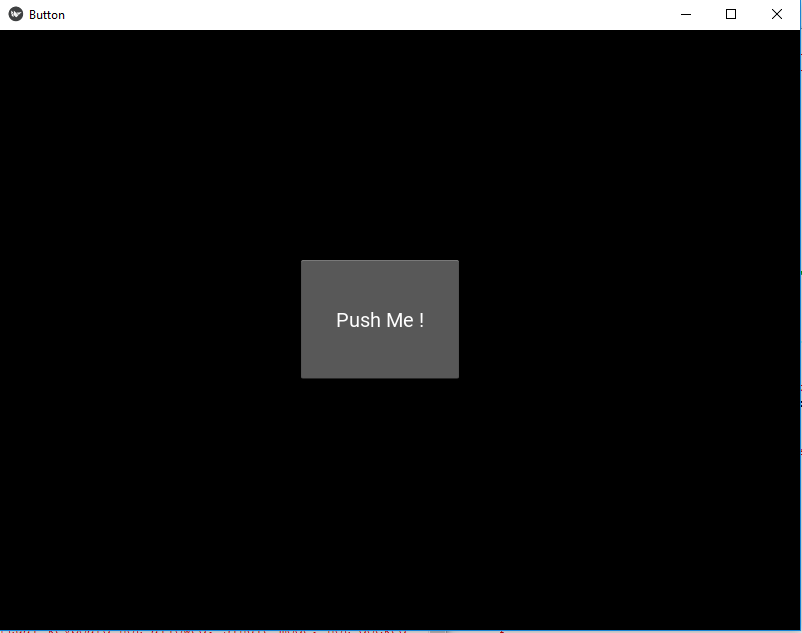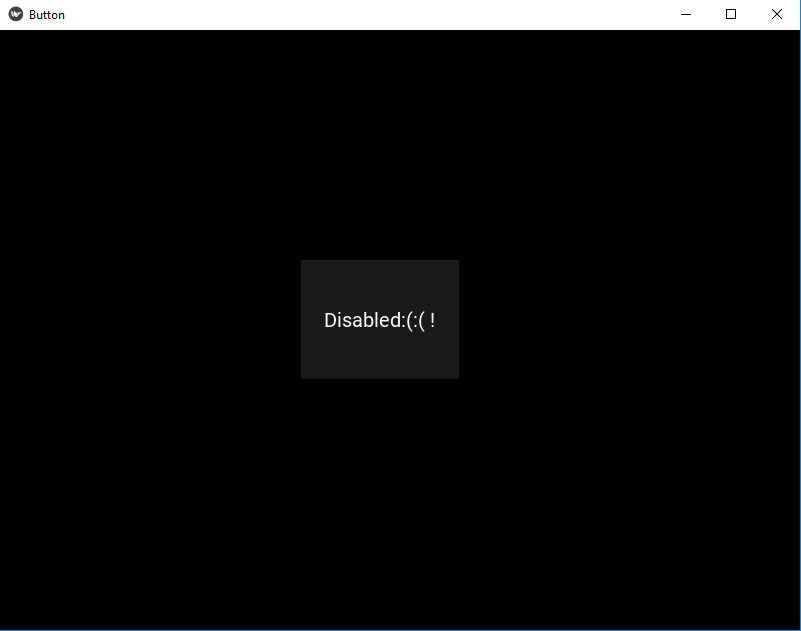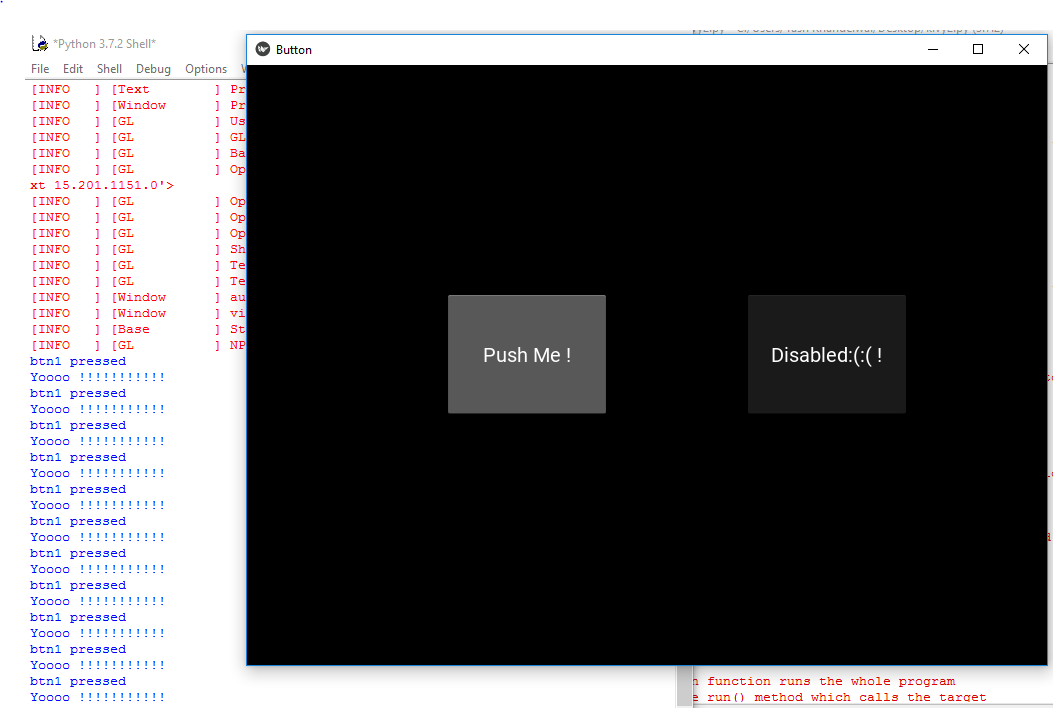Related Articles

# Disable Kivy Button

• Last Updated : 19 Feb, 2020

In this article, we will learn how to disable a button in kivy, there are some places where we need to disable the buttons So in this article you will learn how to do that.

Kivy Tutorial – Learn Kivy with Examples.

The Button is a Label with associated actions that are triggered when the button is pressed (or released after a click/touch). We can add functions behind the button and style the button.But to disable the button we have a property name :

`disabled that must be true`

this property will help in disabling the button i.e. button will be there but is of no use as it is disabled no functionality of button will work.

Note: disabled property was introduced in version 1.8.0. If you want to use it you need to actualize your framework.

Basic Approach to follow while creating and disabling button :

-> import kivy
-> import kivy App
-> import button
-> set minimum version(optional)
-> Extend the class
-> Add and return a button
-> Add disabled = true to disable button
-> Run an instance of the class

Firstly, let’s see how to create a fully working button and then see how to disable it and its functionality.

Code #1: How to create fully working button
 `def` `build(``self``):``    ``# use a (r, g, b, a) tuple``    ``btn ``=` `Button(text ``=``"Push Me !"``,``                 ``font_size ``=``"20sp"``,``                 ``background_color ``=``(``1``, ``1``, ``1``, ``1``),``                 ``color ``=``(``1``, ``1``, ``1``, ``1``),``                 ``size ``=``(``32``, ``32``),``                 ``size_hint ``=``(.``2``, .``2``),``                 ``pos ``=``(``300``, ``250``))`` ` `    ``return` `btn`

Output:Code #2: How to disable the button

 `def` `build(``self``):``        ``# use a (r, g, b, a) tuple``        ``btn ``=` `Button(text ``=``"Push Me !"``,``                     ``font_size ``=``"20sp"``,``                     ``background_color ``=``(``1``, ``4``, ``6``, ``1``),``                     ``color ``=``(``1``, ``1``, ``1``, ``1``),``                     ``size ``=``(``32``, ``32``),``                     ``size_hint ``=``(.``2``, .``2``),``                     ``pos ``=``(``300``, ``250``),`` ` `                     ``# Disabling the button   ``                     ``disabled ``=` `True`` ` `                     ``)`

Output:Code #3: Both disable and working button together

 `# import kivy module ``import` `kivy ``   ` `# this restrict the kivy version i.e ``# below this kivy version you cannot ``# use the app or software ``kivy.require(``"1.9.1"``) ``   ` `# base Class of your App inherits from the App class. ``# app:always refers to the instance of your application ``from` `kivy.app ``import` `App ``   ` `# creates the button in kivy ``# if not imported shows the error ``from` `kivy.uix.button ``import` `Button `` ` `# This layout allows you to set relative coordinates for children. ``from` `kivy.uix.relativelayout ``import` `RelativeLayout `` ` `# class in which we are creating the button ``class` `ButtonApp(App): ``       ` `    ``def` `build(``self``):`` ` `        ``r1 ``=` `RelativeLayout()`` ` `         ` `        ``# working button``        ``btn1 ``=` `Button(text ``=``"Push Me !"``, ``                   ``font_size ``=``"20sp"``, ``                   ``background_color ``=``(``1``, ``1``, ``1``, ``1``), ``                   ``color ``=``(``1``, ``1``, ``1``, ``1``), ``                   ``size ``=``(``32``, ``32``), ``                   ``size_hint ``=``(.``2``, .``2``), ``                   ``pos ``=``(``200``, ``250``)) `` ` `        ``# disabled button``        ``btn2 ``=` `Button(text ``=``"Disabled:(:( !"``, ``                   ``font_size ``=``"20sp"``, ``                   ``background_color ``=``(``1``, ``1``, ``1``, ``1``), ``                   ``color ``=``(``1``, ``1``, ``1``, ``1``), ``                   ``size ``=``(``32``, ``32``), ``                   ``size_hint ``=``(.``2``, .``2``), ``                   ``pos ``=``(``500``, ``250``),`` ` `                   ``# Add disabled property true to disabled button``                   ``disabled ``=` `True``)`` ` `        ``r1.add_widget(btn1)``        ``r1.add_widget(btn2)``        ` `        ``# bind() use to bind the button to function callback ``        ``btn1.bind(on_press ``=` `self``.callback)``        ``return` `r1 ``   ` `    ``# callback function tells when button pressed ``    ``def` `callback(``self``, event): ``        ``print``(``"button pressed"``) ``        ``print``(``'Yoooo !!!!!!!!!!!'``) ``           ` `   ` `# creating the object root for ButtonApp() class  ``root ``=` `ButtonApp() `` ` `# run function runs the whole program ``# i.e run() method which calls the target ``# function passed to the constructor. ``root.run() `

Output:Attention geek! Strengthen your foundations with the Python Programming Foundation Course and learn the basics.

To begin with, your interview preparations Enhance your Data Structures concepts with the Python DS Course. And to begin with your Machine Learning Journey, join the Machine Learning – Basic Level Course

My Personal Notes arrow_drop_up